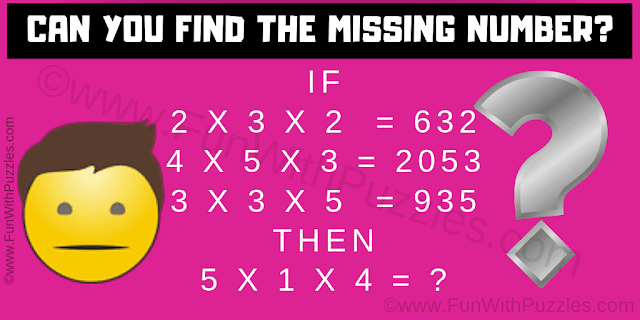This is an easy logic math question for kids which you need to answer as quickly as possible. In this Math Logic Question, you are shown some logical number equations. Decode the logic used in these number equations. Apply the same logic to the last number equation and then find the value of the missing number which replaces question mark.Can you find the value of the missing number?
Answer of this "Quick Logic Math Question for Kids", can be viewed by clicking on the answer button.